# Tests and probability - effects of various factors on test performance

## Idea

One of the primary ways to check a person's knowledge of a subject is a test. Not all tests are created equal though - some actually test knowledge while others test only recitation. For instance, "what was the author's theme in the book" vs "what did the author say on page 5". This is even more subtle in the sciences, such as "calculate the radius of a geosynchronous orbit around the earth" vs "what is the speed of an object accelerated at 2 m/s^2 for 2 seconds", the latter being a formula-recall rather than knowledge question.

Sometimes the "bad" types of tests are administered - where what counts is not true knowledge but word-for-word memorization of material. To spend the least possible effort on those tests while still getting a decent grade, I undertook this analysis.

## Problem Statement

No practical test can cover everything taught in a course, so theoretically not all of the material actually needs to be learned. For instance, if I have studied only 90% of the material, what is my most likely grade on a test, if the test only covers 50% of the course material?

## Solution

As the problem is presented it is rather vague. We make some assumptions:

• There exist N questions that cover everything taught in the course
• The amount of material actually studied can be represented as S, where S/N = empirical measure of "percent of material studied"
• The test cannot have all N questions and instead has T questions, each of which can be answered either correctly or incorrectly
• The questions related to material studied will be answered correctly, while those not studied for will be answered incorrectly
• Correct answer is 1 point and incorrect answer is 0 points contributed towards the grade on the test
• The distribution of material studied and material on the test is random
• Studying information related to all N questions means one is guaranteed to get 100% on the test regardless of T
• Each question covers a single part of material, in other words it can be studied for individually
• Each piece of material learned can have a question related to it on the test

Let's say S/N=90% as in the problem statement, and T/N=10%. Then if I took the test multiple times, what would be my average grade? It will be 90%, since the questions on the test change randomly every time and in an infinite series will effectively represent the entirety of N. In fact, regardless of the size of the test, that is even if T/N=1%, on average my grade would be 90%. Seems like there is no way to get any grade inflation here. This may be surprising if you believe that a one-question exam is more difficult than a twenty-question exam. A one-question exam in fact fairly represents how well one has studied but only on average, that is when it is taken multiple times and the scores are averaged. That effectively gets rid of the one-time nature of the exam. Thus, a better approach to the problem is needed.

The better approach lies in finding the probability distribution of attaining various grades. As mentioned, with any value of T/N the averaged final grade over multiple tests will represent S/N, however the results on each individual test will clearly depend on T/N. At this point the variables need to take on a numeric value. Let N=100 word definitions for a language test (define this word: ___), S=90 word definitions learned. If T=100 questions on the test, the only possible grade is 90%. If T=10 though, it is possible to achieve 100% (even with 90% knowledge) or on the contrary 0%.

Let G be the number of questions answered correctly on the test (G<=T) and P(G) be the probability of answering G questions on a random test of T questions out of N possible questions.
Then clearly ∫ P(G) dG [0, T] = 1, stating that P(G) is a probability distribution representing all valid grades.
It follows that ∫ G/T*P(G) dG [0, T] = S/N, that is the average grade will be S/N or 90% in the given example. This is a restatement of the first paragraph.
What I am interested in here is rather than finding the numeric integral to find a representation of P(G) and create a table or chart based on that.

This is easier to analyze using combinations rather than probabilities. The number of possible tests of size T is C(N, T) . The number of ways of getting exactly G points on such a test is C(S, G) * C(N-S, T-G).
Thus P(G) = C(S, G) * C(N-S, T-G) / C(N, T)

## Graphical analysis

Now, it is possible to use P(G) for various grades and analyze the correlation between amount of material studied, length of test, and the scope of the course (number of possible questions asked). The following images represent multiple cases which demonstrate the trends associated with changing each of these variables. They have been used with specific numeric values of N and S, which in the real world is not quantifiable, but the analysis is still valid as it represents effects of practical changes, such as increasing N or S (which is viable in a real-world situation). Thus the trends portrayed in the graphs below are more important than specific number values.

### Effects of studying more material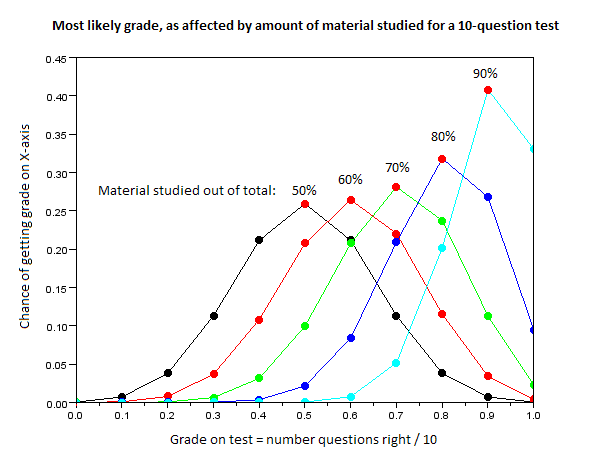Here it is evident that studying more material increases the probability of attaining a grade representative of the amount of material studied. Note that the chance of obtaining a grade of 100% increases non-linearly with increasing amount of material studied (one is more than three times as likely to get 100% after studying 90% of material than after studying 80%)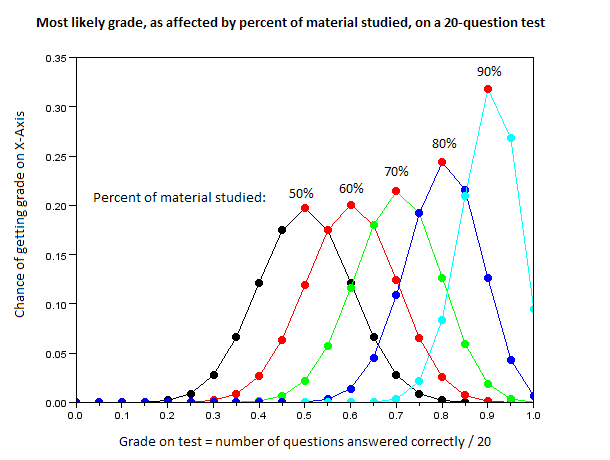Same concept as above, but now the test has twice as many questions. Notice that the peaks get narrower - thus the longer test is a more accurate representation of the true amount of material studied. While the chance of 100% still increases nonlinearly, it is much smaller than the same chance on the shorter test above.

### Effects of test length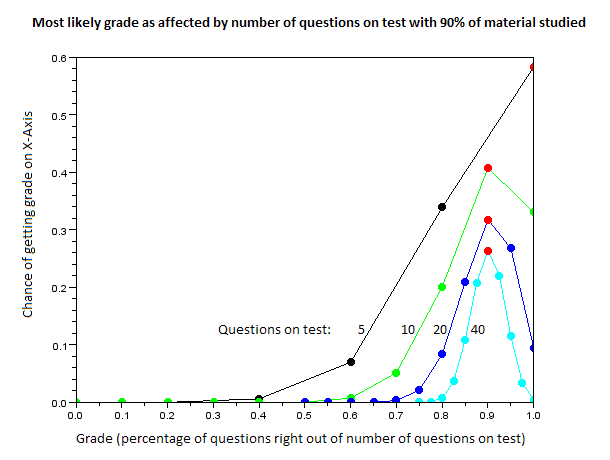This graph is an overlay of probability distributions for different test lengths, for each of which 90% of material has been studied. Notice that this does not violate the distribution function rule (integral=1) since the curves for tests with many questions have more possible grades than tests with fewer questions, since grades have been quantized to integers and the X-axis is fractions that are scaled by T. The important feature to notice here is that more questions non-linearly decreases the chance for both a higher and a lower grade than the one that should be attained based on amount of material studied. The increased accuracy of testing true knowledge with longer tests is alluded to above. Finally, notice that there is a very small region in which a grade higher than studied for is actually feasible - having even ten different questions is enough for an accurate indicator of knowledge, while five is questionable.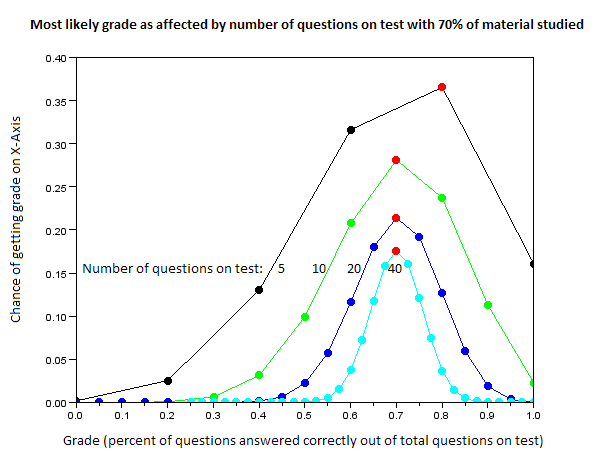Same idea as above, except this time only 70% of material has been studied rather than 90%. The trends described above still stand.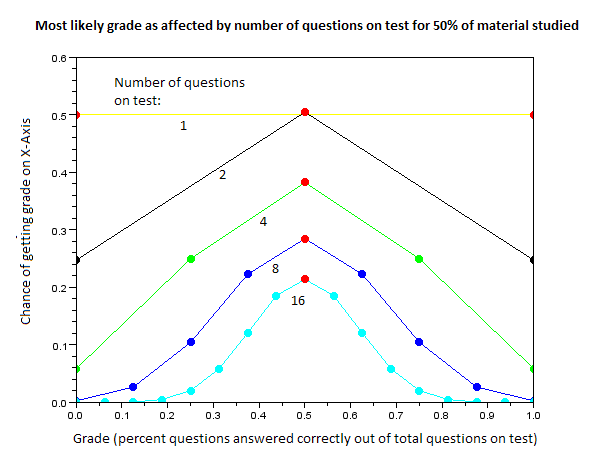It may seem that if the test only covers a small (<10%) portion of the material learned during the course it may be practical to get away with studying only 50% of material. This graph shows that this is clearly not the case, as even with two questions the most likely grade is a 50% and it gets only more likely with increasing questions.

### Boundary test length conditions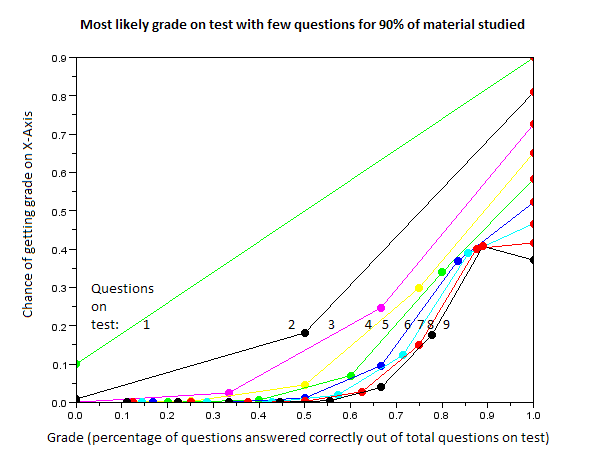This represents the grade distribution for 90% of material studied on tests with few questions (less than ten). In all of them except 9, the most likely grade is 100% - higher than amount studied, simply due to the quantized nature of the grading. The chances of receiving a lower grade than 90% are also fairly high, about 40% for a test with 7 to 9 questions (this is balanced out by the fact that the grade is close to 90% - yet it is still not the most valuable as an accurate indicator, and certainly the difference between A and B can be felt by the student). In fact, with 9 questions the student who studied at an A level (90%) is most likely to get a B (88.9%)! My advice for teachers is to use at least ten questions, then this issue does not arise (since 1 question lost on 10-question test is 9/10=90% while 8/9=88.9%). Again, not much difference mathematically but in terms of self esteem would you rather get a low A or a high B?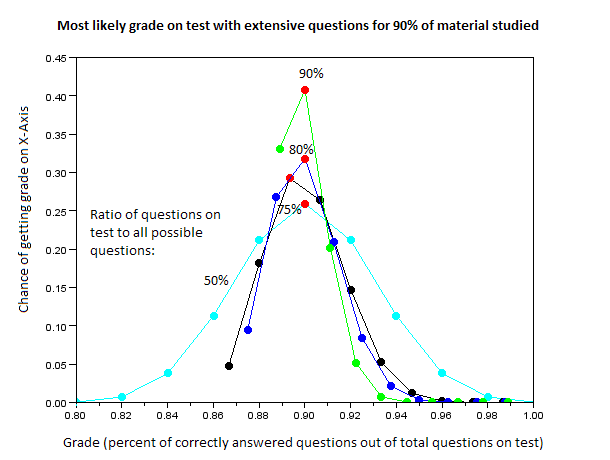This represents the grade distribution for 90% of material studied on a test with extensive questions, covering a significant percentage of all material studied in the course (50% and more). As is evident, the grades are much more representative of actual effort (the X-axis starts at 80%, unlike above graph starting at 0%). It is still possible to get a grade of less than 90% if an odd number of questions is used on the test (represented by 75% test size case). With increasing test length, grades much lower than studied for are mathematically unattainable, while those much higher are realistically unattainable (this is inverted when very little material is studied), thus a longer test is a better indicator of amount of content learned.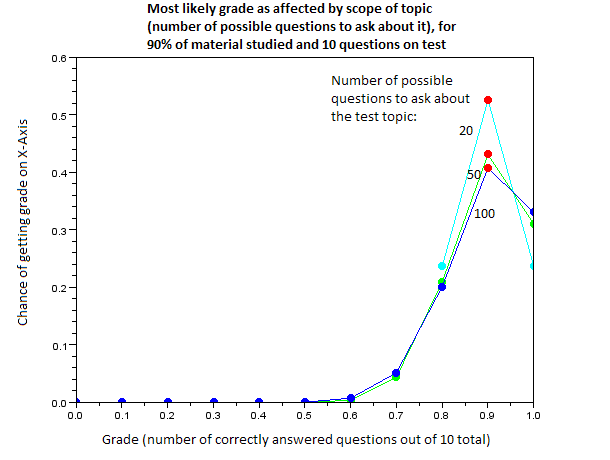In this graph, each test contains 10 questions and 90% of material has been studied; however the constant describing the breadth of the course (the amount of unique questions that can be asked) is changed from 20 to 100. With an increasing number of questions, the test becomes less likely to accurately indicate the amount of material studied. Particularly, the chance of attaining a higher grade becomes more attainable, as does the probability of getting a much lower grade. The chance of getting a representative grade meanwhile decreases.
In realistic terms this is due to luck in the types of questions that appear on the test taking over the effects of the test-taker's knowledge of full subject matter, since full knowledge is impractical for a very broad topic.Thus it is better to give brief and focused tests than wide-spanning and general tests if one is seeking how much the test taker has actually studied.

## Conclusion

Here are my conclusions after this analysis. In hindsight, some of these seem obvious, although some are quite interesting. However, it is always nice to have mathematical basis, which all of these claims now do.

• The most likely grade one is to receive will be reflective of the amount of material studied in all cases
• The more questions on the test, the more closely the test represents how much one has actually studied
• The more focused the subject matter of the test, the more closely the test represents how much one has actually studied
• The less questions on the test, the more likely it is for one to obtain a higher grade than studied for (on the other hand, the more probable it is that one will obtain a lower grade than studied for)
• The more material one has studied, the smaller the relative chance of getting a higher-than-expected grade (on the other hand, the chance is relatively greater if one has studied less material)
• To have a practical chance of obtaining a grade higher than studied for, the number of questions on the test should be less than 10
• Having nine questions on a test will create more high-B grades as opposed to ten questions which would create instead low-A grades
• Thus a 9-question test is the worst thing a teacher could do for students' self esteem (since with 8 questions there is a higher relative chance for 100% while with 10 there is a way to get 90%)

A brief summary:
For students - studying 50% will with near-certainty yield a grade of 50% as long as the test is more than one question
For instructors - a test with 10 or more questions that is focused on a specific subject matter is the most likely to accurately represent the knowledge of the test-taker